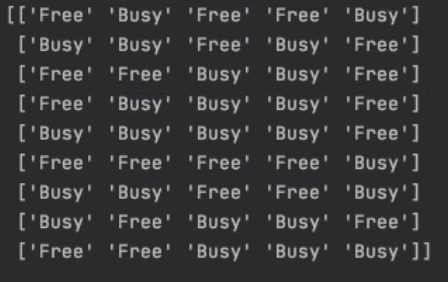# What is the ‘python version’ of array[i]?

I am learning Python and trying to compare the elements of multiple arrays/lists at a time, my arrays are Numpy arrays (I guess?) as I did the following to create them and the answer mentions the output as a numpy array

I have 3 arrays of length 40 in total and what I want to do is iterate through them and check if elements at the same index are equal, in a programming language such as Java I can easily do this using something like:

``````for (int i=0;i<40; i++)
if(array1[i]==array2[i]==array3[i]
//do whatever
``````

however I am having trouble doing this as tutorials on iterating through the array will be something like this:

``````for x in arr:
\\do work
``````

with no reference to an index, any help would be appreciated

UPDATE: for more clarification, I used the linked answer to import a csv file with strings to a numpy array, so my array elements are strings

here is what print(array) outputs, I have 3 of those